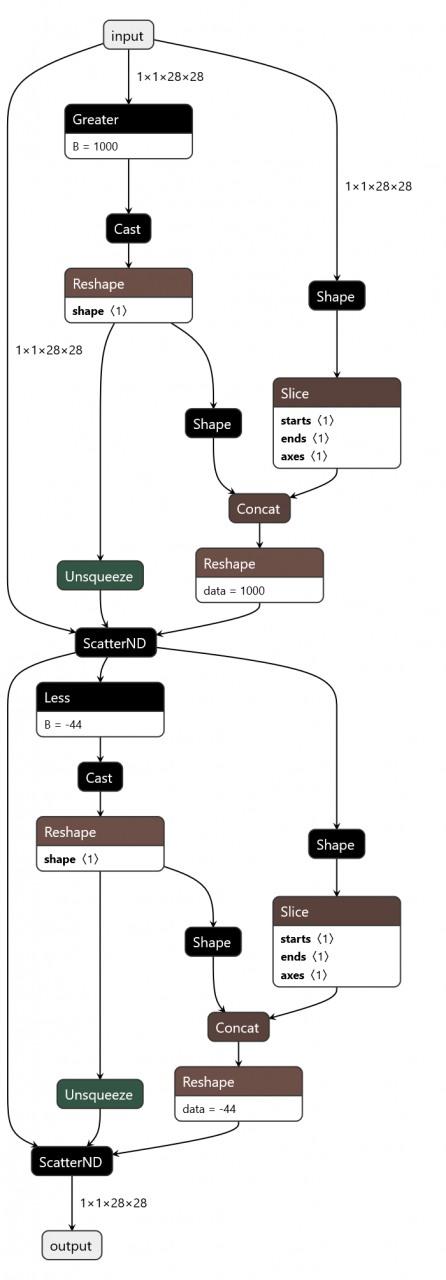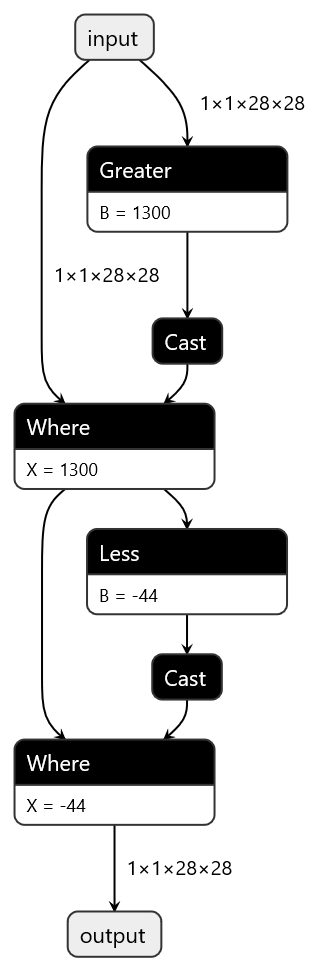Project scenario:

The python model is converted to onnx model and can be exported successfully, but the following errors occur when loading the model using onnxruntime

InvalidGraph: [ONNXRuntimeError] : 10 : INVALID_ GRAPH : Load model from T.onnx failed:This is an invalid model. Type Error: Type ‘tensor(bool)’ of input parameter (8) of operator (ScatterND) in node (ScatterND_ 15) is invalid.

Problem Description:

``````import torch
import torch.nn as nn
import onnxruntime
from torch.onnx import export

class Preprocess(nn.Module):
def __init__(self):
super().__init__()
self.max = 1000
self.min = -44

def forward(self, inputs):
inputs[inputs>self.max] = self.max
inputs[inputs<self.min] = self.min
return inputs

x = torch.randint(-1024,3071,(1,1,28,28))
model = Preprocess()
model.eval()

export(
model,
x,
"test.onnx",
input_names=["input"],
output_names=["output"],
opset_version=11,
)

session = onnxruntime.InferenceSession("test.onnx")
``````

Cause analysis:

The same problem can be found in GitHub of pytorch #34054

Solution:

The specific operations are as follows: Mr. Cheng ` mask `, and then use ` torch.masked_ Fill() ` operation. Instead of using the index to directly assign the entered ` tensor `

``````class MaskHigh(nn.Module):
def __init__(self, val):
super().__init__()
self.val = val

def forward(self, inputs):
x = inputs.clone()
return output

def __init__(self, val):
super().__init__()
self.val = val

def forward(self, inputs):
x = inputs.clone()
return output

class Clip(nn.Module):
def __init__(self):
super().__init__()

def forward(self, inputs):
output = self.high(inputs)
output = self.low(output)
return output
``````

Netron can be used to visualize the calculation diagram generated by the front and rear methodsIndex assignment